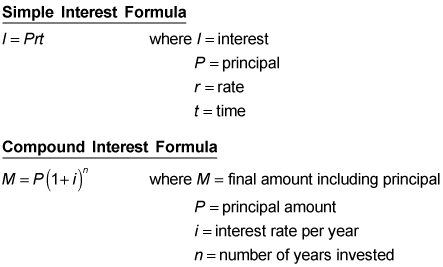##### GED Test 2022 / 2023 For Dummies with Online PracticeIf you really enjoy tackling interest rate problems (and who doesn't?), then you should have no problem solving the ones you encounter on the GED Math test. As you'll see in the following practice questions, they're pretty straightforward—you just need to be comfortable with using the simple and compound interest formulas.

The GED Math test includes a formula sheet, but here are the simple and compound interest formulas for your reference:## Practice questions

1. If Giorgio borrows \$100 for one year and three months and repays \$108 including simple interest, what rate of interest was he charged?

A. 6.4% B. 8.0% C. 4.0% D. 4.6%

2. The formula for compound interest on a loan is M = P (1 + i)n, where M is the final amount including the principal, P is the principal amount, i is the rate of interest per year, and n is the number of years invested.

If Amy invested the \$1,574 she got as gifts for graduating from elementary school in a Guaranteed Investment Certificate, paying 3.75 percent compound interest calculated annually for the seven years until she graduates from college, how much money would she have?

A. \$1,978.18 B. \$462.67 C. \$2,036.67 D. \$1,878.18

1. The correct answer is Choice (A).

This question tests your ability to evaluate an answer by using a formula. This formula, I = prt, isn't in the format you want because you want to calculate the rate, which means solving for r. You can change the equation to

which allows you to calculate the rate from the information given. Substituting into this equation, you get

(Remember that 1 year and 3 months is 1 1⁄4, or 1.25 of a year.)

2. The correct answer is Choice (C). Using the formula given on the formula sheet, you can calculate the total amount Amy would have at the end of seven years: